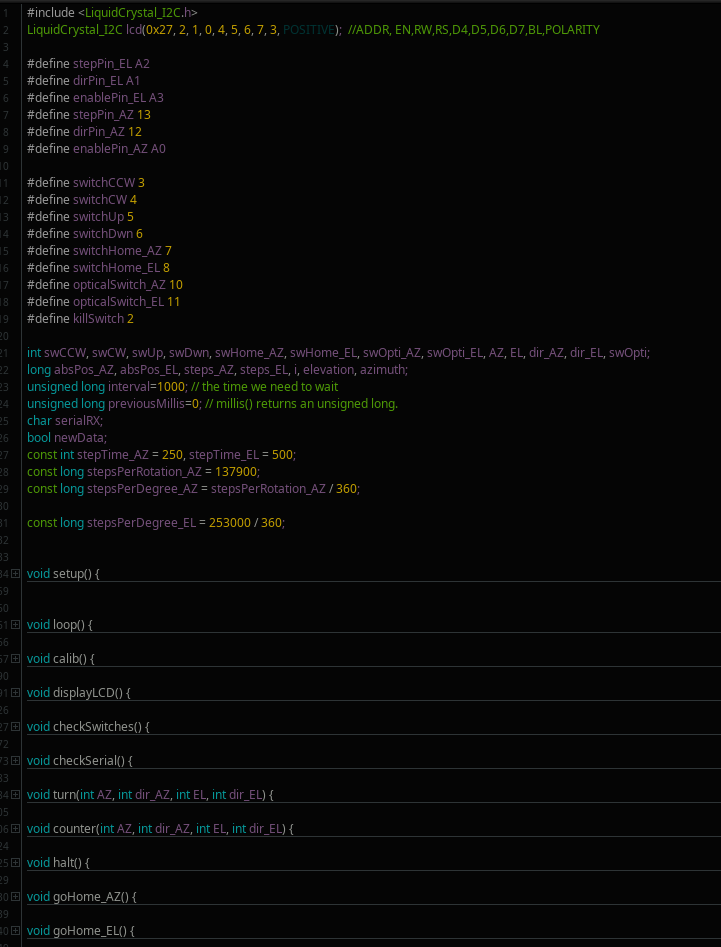## Arduino code

For the impatient: link to the whole sketch for you to download (version May 21, 2021). There might be some extra stuff in there, like a calibration function or the beginning of a serial function, but you can safely ignore those, or study them at your own leisure.

When I started writing this sketch I wanted it to be as simple and flexible as possible and avoid redundancy, so everything is put into functions and no libraries used, except for the LCD. Apart from the setup() and loop() functions the most important one is turn(), which makes the stepper motors move. This function takes four integers (HIGH or LOW) two to turn the steppers on and two for the direction. With these values it either turns one or both of the steppers in a certain direction, then passes the values on to the counter() function.

```void turn(int AZ, int dir_AZ, int EL, int dir_EL) {
digitalWrite(enablePin_AZ,!AZ);          //if AZ is set to 0 the enable pin of the TMC2808 will become active LOW and azimuth rotation is executed, this is done by the inverse of AZ, hence !AZ
digitalWrite(enablePin_EL,!EL);          //if EL is set to 0 the enable pin of the TMC2808 will become active LOW and elevation rotation is executed, this is done by the inverse of EL, hence !EL
digitalWrite(dirPin_AZ,dir_AZ);
digitalWrite(dirPin_EL,dir_EL);
digitalWrite(stepPin_AZ,HIGH);
digitalWrite(stepPin_EL,HIGH);
digitalWrite(stepPin_AZ,LOW);
digitalWrite(stepPin_EL,LOW);

if(AZ == HIGH && EL == LOW) {
delayMicroseconds(stepTime_AZ);
}

if(EL == HIGH && AZ == LOW) {
delayMicroseconds(stepTime_EL);
} else {delayMicroseconds(stepTime_AZ);}

counter(AZ, dir_AZ, EL, dir_EL);

} //end void turn```

The counter() function simply records the absolute position of the stepper motor, which defaults to 0 when the sketch is loaded. Therefore homing of both AZ and EL is required.

```void counter(int AZ, int dir_AZ, int EL, int dir_EL) {
if(AZ == HIGH && dir_AZ == 0) {
absPos_AZ++;
}
if(AZ == HIGH && dir_AZ == 1) {
absPos_AZ--;
}
if(EL == HIGH && dir_EL == 0) {
absPos_EL--;
}
if(EL == HIGH && dir_EL == 1) {
absPos_EL++;
}
} //end void counter```

The halt() function simply makes the enable pins of the TMC2808 become HIGH, so the motors won't have any current going through them.

```void halt() {
digitalWrite(enablePin_AZ,HIGH); //for the TMC2208 the enable pin has to be set HIGH for the driver to be disengaged
digitalWrite(enablePin_EL,HIGH);
}```

The main operation of the rotator is with 4 momentary push switches, two for azimuth, two for elevation. There are also two momentary home switches to bring the rotator to back to 0 degrees azimuth and elevation. These home buttons call the goHome_AZ() and goHome_EL() functions, the other buttons can be used in a variety of combinations. A combination of one azimuth and one elevation switch is allowed, but not two azimuth or two elevation switches. The halt() function called when no switch has been activated.

```void checkSwitches() {

//these if-statements are used when a single push button is pressed. The rotator goes either up or down, CW or CCW
if(swCCW == HIGH && swCW == LOW && swUp == LOW && swDwn == LOW) {turn(1,1,0,0); }
if(swCCW == LOW && swCW == HIGH && swUp == LOW && swDwn == LOW) {turn(1,0,0,0); }
if(swCCW == LOW && swCW == LOW && swUp == HIGH && swDwn == LOW) {turn(0,0,1,1); }
if(swCCW == LOW && swCW == LOW && swUp == LOW && swDwn == HIGH) {turn(0,0,1,0); }

//this prevents any action when two push buttons are pressed at once
if(swCCW == HIGH && swCW == HIGH && swUp == LOW && swDwn == LOW) {turn(0,0,0,0); }
if(swCCW == LOW && swCW == LOW && swUp == HIGH && swDwn == HIGH) {turn(0,0,0,0); }

//these if-statements are used when a combination of up/down and CW/CCW are pressed
if(swCCW == HIGH && swCW == LOW && swUp == HIGH && swDwn == LOW) {turn(1,1,1,1); }
if(swCCW == HIGH && swCW == LOW && swUp == LOW && swDwn == HIGH) {turn(1,1,1,0); }
if(swCCW == LOW && swCW == HIGH && swUp == HIGH && swDwn == LOW) {turn(1,0,1,1); }
if(swCCW == LOW && swCW == HIGH && swUp == LOW && swDwn == HIGH) {turn(1,0,1,0); }

if(swHome_AZ == HIGH) {
goHome_AZ();
}

if(swHome_EL == HIGH) {
goHome_EL();
} else {
halt();
}
} //end void checkSwitches```

When the homing switches are activated they trigger either one of these functions. The rotator will turn one way until the slotted IR photo sensor is triggered and becomes high. The absolute positions will be reset to zero then.

```void goHome_AZ() {
do {
turn(1,0,0,0);
displayLCD();
} while(swOpti_AZ == LOW);

absPos_AZ = 0;
} //end void goHome_AZ

void goHome_EL() {
do {
turn(0,0,1,0);
displayLCD();
} while(swOpti_EL == LOW);

absPos_EL = 0;
} //end void goHome_EL```

The azimuth and elevation are displayed on a 16x2 character LCD and for this I use a library (the only one used in this sketch). I tried to use a timing based solution with millis() where the display is updated every second. This works, but when the steppers are activated it will still interrupt them while turning and a "thump" can be heard every second.

```void displayLCD() {
if((unsigned long)(millis() - previousMillis) >= interval) {
azimuth = absPos_AZ / stepsPerDegree_AZ;
if(absPos_AZ <= 0) {azimuth = 360 + azimuth;};
if(azimuth == 360) {azimuth = 0;};
elevation = absPos_EL / stepsPerDegree_EL;
lcd.clear();
lcd.setCursor(0, 0);
lcd.print("AZ: ");
lcd.setCursor(4, 0);
lcd.print(azimuth);
lcd.setCursor(9, 0);
lcd.print("EL: ");
lcd.setCursor(13, 0);
lcd.print(elevation);
lcd.setCursor(0,1);
lcd.print(absPos_AZ);
previousMillis = millis();  // save the "current" time
}
}```

So with all these functions to control the steppers the loop() function simply looks like this:

```void loop() {
checkSwitches();
displayLCD();
} //end void loop```

And the outline of the whole sketch:The code highlighter I used: https://highlight.hohli.com/index.php

Page 1: Materials used

Page 2: Photos and Videos

Page 4: Code for testing purposes